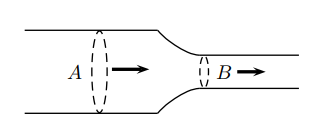# Problem: An incompressible fluid is flowing through a horizontal tube which, at some point has a constriction such that the area of the tube becomes much smaller. How do the fluid pressure and speed of flow compare at point B in the constricted region to their values at point A in the normal part of the tube? A. The pressure and speed of flow are both much greater at point A than at point B. B. The pressure and speed of flow are both much greater at point B than at point A. C. At point B, the speed of flow is less but the pressure is greater than at point A. D. Because the fluid is incompressible, the pressure and speed of flow must be constant throughout the tube. E. At point A, the speed of flow is less but the pressure is greater than at point B.

79% (23 ratings)
###### Problem Details

An incompressible fluid is flowing through a horizontal tube which, at some point has a constriction such that the area of the tube becomes much smaller.

How do the fluid pressure and speed of flow compare at point B in the constricted region to their values at point A in the normal part of the tube?

A. The pressure and speed of flow are both much greater at point A than at point B.

B. The pressure and speed of flow are both much greater at point B than at point A.

C. At point B, the speed of flow is less but the pressure is greater than at point A.

D. Because the fluid is incompressible, the pressure and speed of flow must be constant throughout the tube.

E. At point A, the speed of flow is less but the pressure is greater than at point B.